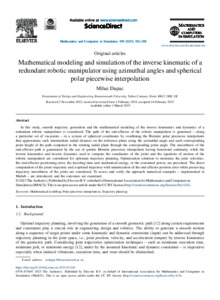# Mathematical modeling and simulation of the inverse kinematic of a redundant robotic manipulator using azimuthal angles and spherical polar piecewise interpolation.

Dupac, M., 2023. Mathematical modeling and simulation of the inverse kinematic of a redundant robotic manipulator using azimuthal angles and spherical polar piecewise interpolation. Mathematics and Computers in Simulation, 209, 282-298.

Full text available as:Preview
PDF (OPEN ACCESS ARTICLE)
1-s2.0-S0378475423000836-main.pdf - Published Version

2MB

## Abstract

In this study, smooth trajectory generation and the mathematical modeling of the inverse kinematics and dynamics of a redundant robotic manipulator is considered. The path of the end-effector of the robotic manipulator is generated – along a particular set of via-points – in a system of spherical coordinates by combining the Hermite polar piecewise interpolants that approximate each intermediate radial distance on the reference plane using the azimuthal angle and each corresponding point height of the path computed in the rotating radial plane through the corresponding polar angle. The smoothness of the end-effector path is guaranteed by the use of quintic Hermite piecewise interpolants having functional continuity, while the inverse kinematics and inverse dynamics used to compute the coordinates of the joints and the equations of motion of the robotic manipulator are considered for optimum trajectory planning. Optimization solutions to minimize the joint velocities, the end-effector positioning error, the traveling time and mechanical energy, or the consumed power, are presented. The direct computation of the joints trajectory and a optimal trajectory with minimization of the end-effector position error while preserving trajectory smoothness of the joints are considered. To assess and verify the approach numerical examples – implemented in Matlab – are introduced, and the outcomes are examined.

Item Type: Article 0378-4754 Mathematical modeling; Polar piecewise interpolation; Trajectory planning Faculty of Science & Technology 38328 Symplectic RT2 15 Mar 2023 14:03 15 Mar 2023 14:03